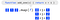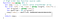# Higher-order Functions In JavaScript

## A higher-order function is a function that either *takes a function as one of its parameters or *returns another function. Why you would want to do this will become more clear as you explore the logic of a higher-order function. So, let’s take a look.

Here’s a list of my best web development tutorials.

Complete CSS flex tutorial on Hashnode.

Ultimate CSS grid tutorial on Hashnode.

Higher-order functions .map, .filter & .reduce on Hashnode.

The first time I heard about higher-order functions I couldn’t wrap my mind around what it actually meant and why they are called this way.

You are probably already familiar with regular functions in JavaScript. They are usually referred to as first-order functions. But they usually never take a function as one of its parameters, nor return another function.

But why would we even want to pass or return a function from another function? This can actually be quite useful for writing compact code.

Higher-order functions are often used in context of Functional Programming style. FP style has roots in calculus. (Or more specifically lambda calculus.)

Functional in FP doesn’t refer to JavaScript functions but math ones.

Higher-order functions can shorten your code by making it look more like a math function. This has several benefits: clean code, and just general level awesomeness of thinking of your code as a math equation.

Especially the type of code you would write by using for-loops instead.

Most for-loops you can possibly write accomplish relatively the same things, with just a few differences. Mapping a value to another value might intersect with the logic of filtering values based on some condition.

Developers of JavaScript language added a few helper functions that cover most of those cases. If you learn how to use them you’ll probably never have to write another for-loop in your life.

# Array.map

The Array.map method is one of the most common higher-order functions. We will use it as an example here. But there are several other higher-order functions: .filter, .reduce, etc.

Array.map method takes a function to run on every item in the array. Then it returns a modified copy of the original array.

Here I created add_one function to pass into map function:Array.map is a natively built-in function that exists on all Array objects.

To see how it all works, first, let’s define an initial set of values:

Now, let’s create the function that will modify the values.

The body of the function depends on what type of modifications you’re looking to apply to each array item.

In this example we will create a function called add_one, which simply adds 1 to the value. This is not a higher-order function, just a helper.

JavaScript already provides a set of high-order functions. But why not write our own just to understand how they work?

Writing our own higher-order function

For a function to qualify as a higher-order function it either needs to take a function as one of its parameters, or return a function. As long as one of those conditions is fulfilled, we are creating a higher-order function.

The map function will take an array to work on. It will return a copy of that array with each item modified by the add_one function we have written earlier, which will be passed as the second parameter.

Let’s write our own version of map which does exactly what Array.map does.Source code of a higher-order function map. It is assumed that the array will contain only numeric values.

The function map takes two parameters: an arbitrary array of values and the function which we want to apply to each item in the array.

First, copy array was created and assigned to an empty array literal: []. This array will store a modified copy of the original array that was passed into the function.

Then a for loop iterates through the array we received as first parameter.

Once all items have been copied and processed by add_one function, they will be stored in copy array which will then be used as the return value of the function.

Just as a side note: an accumulator in another higher-order function Array.reduce serves a similar purpose as copy array in this example.

Let’s try out our map function in action:

As you can see it does exactly what Array.map does behind the scenes. Let’s increment the values again on the returned array, this time using the built in map method:

This operation is so common that it was added as a native method to the Array object. Instead of writing your own code always try to figure out if there is a function that already exists.

Chances are, it has a cleaner and more performance-efficient implementation than what you would write yourself using for-loops.

Written by